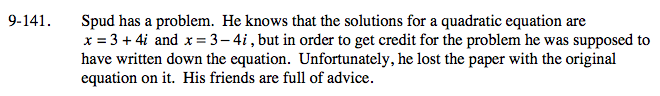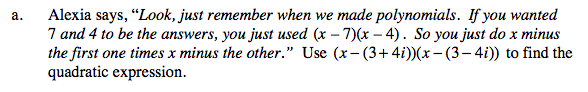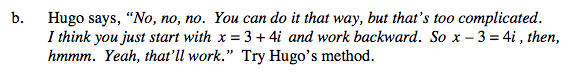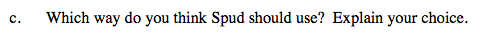### Home > A2C > Chapter 9 > Lesson 9.3.2 > Problem9-141

9-141.
1. Spud has a problem. He knows that the solutions for a quadratic equation are x = 3 + 4i and x = 3 − 4i, but in order to get credit for the problem he was supposed to have written down the equation. Unfortunately, he lost the paper with the original equation on it. His friends are full of advice. Homework Help ✎

1. Alexia says, “Look, just remember when we made polynomials. If you wanted 7 and 4 to be the answers, you just used (x − 7)(x − 4) . So you just do x minus the first one times x minus the other.” Use (x − (3 + 4i))(x − (3 − 4i)) to find the quadratic expression.

2. Hugo says, “No, no, no. You can do it that way, but that's too complicated. I think you just start with x = 3 + 4i and work backward. So x − 3 = 4i, then, hmmm. Yeah, that'll work.” Try Hugo's method.

3. Which way do you think Spud should use? Explain your choice.Use a generic rectangle.

x2 − 6x + 25(x − 3) = (4i)2Which do you think is more efficient? Which helps you understand the most?